• 目录创建一个节点边查看上点和边的信息的属性设置点的属性设置边的属性设置不同类型的（有向Directed graphs , 重边 Multigraphs）的遍历图生成上的一些操作上分析的绘制1. 创建一个import ...
目录
创建一个图
节点
边
查看图上点和边的信息
图的属性设置
点的属性设置
边的属性设置
不同类型的图（有向图Directed graphs , 重边图 Multigraphs）
图的遍历
图生成和图上的一些操作
图上分析
图的绘制
1. 创建一个图
import networkx as nx
g = nx.Graph()
g.clear() #将图上元素清空
所有的构建复杂网络图的操作基本都围绕这个g来执行。
2. 节点
节点的名字可以是任意数据类型的，添加一个节点是
添加一组节点，就是提前构建好了一个节点列表，将其一次性加进来，这跟后边加边的操作是具有一致性的。
or
a = [2,3]
g.nodes() #可以将以上5个节点打印出来看看
加一组从0开始的连续数字的节点
H = nx.path_graph(10)
删除节点
与添加节点同理
g.remove_node(node_name)
g.remove_nodes_from(nodes_list)
3. 边
边是由对应节点的名字的元组组成，加一条边
e = (2,3)
加一组边
通过nx.path_graph(n)加一系列连续的边
n = 10
H = nx.path_graph(n)
删除边
同理添加边的操作
g.remove_edge(edge)
g.remove_edges_from(edges_list)
4. 查看图上点和边的信息
g.number_of_nodes() #查看点的数量
g.number_of_edges() #查看边的数量
g.nodes() #返回所有点的信息(list)
g.edges() #返回所有边的信息(list中每个元素是一个tuple)
g.neighbors(1) #所有与1这个点相连的点的信息以列表的形式返回
g #查看所有与1相连的边的属性，格式输出：{0: {}, 2: {}} 表示1和0相连的边没有设置任何属性（也就是{}没有信息），同理1和2相连的边也没有任何属性
method
explanation
Return True if the graph contains the node n.
Return True if n is a node, False otherwise.
Return True if the edge (u,v) is in the graph.
Return the number of nodes in the graph.
Return the number of nodes in the graph.
Return the number of nodes.
Graph.degree([nbunch, weight])
Return the degree of a node or nodes.
Graph.degree_iter([nbunch, weight])
Return an iterator for (node, degree).
Graph.size([weight])
Return the number of edges.
Return the number of edges between two nodes.
Return a list of nodes with self loops.
Return a list of selfloop edges.
Return the number of selfloop edges.
5. 图的属性设置
为图赋予初始属性
g = nx.Graph(day="Monday")
g.graph # {'day': 'Monday'}
修改图的属性
g.graph['day'] = 'Tuesday'
g.graph # {'day': 'Tuesday'}
6. 点的属性设置
print g.node['benz'] # {'fuel': '1.5L', 'money': 10000}
print g.node['benz']['money'] # 10000
print g.nodes(data=True) # data默认false就是不输出属性信息，修改为true，会将节点名字和属性信息一起输出
7. 边的属性设置
通过上文中对g的介绍可知边的属性在{}中显示出来，我们可以根据这个秀改变的属性
g.clear()
n = 10
H = nx.path_graph(n)
g['color'] = 'blue'
g['weight'] = 4.7
g.edge['weight'] = 4
8. 不同类型的图（有向图Directed graphs , 重边图 Multigraphs）
Directed graphs
DG = nx.DiGraph()
print DG.out_degree(1) # 打印结果：2 表示：找到1的出度
print DG.out_degree(1, weight='weight') # 打印结果：0.8 表示：从1出去的边的权值和，这里权值是以weight属性值作为标准，如果你有一个money属性，那么也可以修改为weight='money'，那么结果就是对money求和了
print DG.successors(1) # [2,4] 表示1的后继节点有2和4
print DG.predecessors(1) #  表示只有一个节点3有指向1的连边
​
Multigraphs
简答从字面上理解就是这种复杂网络图允许你相同节点之间允许出现重边
MG=nx.MultiGraph()
print MG.degree(weight='weight') # {1: 1.25, 2: 1.75, 3: 0.5}
GG=nx.Graph()
for nbr,edict in nbrs.items():
minvalue=min([d['weight'] for d in edict.values()])
print nx.shortest_path(GG,1,3) # [1, 2, 3]
9. 图的遍历
g = nx.Graph()
print n, nbrs
for nbr,eattr in nbrs.items():
# nbr表示跟n连接的点，eattr表示这两个点连边的属性集合，这里只设置了weight，如果你还设置了color，那么就可以通过eattr['color']访问到对应的color元素
data=eattr['weight']
if data<0.5: print('(%d, %d, %.3f)' % (n,nbr,data))
10. 图生成和图上的一些操作
下方的这些操作都是在networkx包内的方法
subgraph(G, nbunch) - induce subgraph of G on nodes in nbunch
union(G1,G2) - graph union
disjoint_union(G1,G2) - graph union assuming all nodes are different
cartesian_product(G1,G2) - return Cartesian product graph
compose(G1,G2) - combine graphs identifying nodes common to both
complement(G) - graph complement
create_empty_copy(G) - return an empty copy of the same graph class
convert_to_undirected(G) - return an undirected representation of G
convert_to_directed(G) - return a directed representation of G
11. 图上分析
g = nx.Graph()
nx.connected_components(g) # [[1, 2, 3], ['spam']] 表示返回g上的不同连通块
sorted(nx.degree(g).values())
通过构建权值图，可以直接快速利用dijkstra_path()接口计算最短路程
>>> G=nx.Graph()
>>> e=[('a','b',0.3),('b','c',0.9),('a','c',0.5),('c','d',1.2)]
>>> print(nx.dijkstra_path(G,'a','d'))
['a', 'c', 'd']
12. 图的绘制
下面是4种图的构造方法，选择其中一个
nx.draw(g)
nx.draw_random(g) #点随机分布
nx.draw_circular(g) #点的分布形成一个环
nx.draw_spectral(g)
最后将图形表现出来
import matplotlib.pyplot as plt
plt.show()
将图片保存到下来
nx.draw(g)
plt.savefig("path.png")
修改节点颜色，边的颜色
g = nx.cubical_graph()
nx.draw(g, pos=nx.spectral_layout(g), nodecolor='r', edge_color='b')
plt.show()
13. 图形种类的选择
Graph Type
NetworkX Class
简单无向图
Graph()
简单有向图
DiGraph()
有自环
Grap(),DiGraph()
有重边
MultiGraph(), MultiDiGraph()
参考资源
展开全文• 点击上方蓝字关注我们 最近发现一个特别好用的python库，能够绘制精美的关系图，俗话说有好东西要学会分享，所以袁厨就肝了这篇文章，大家可以参考一下。一、概述 Networ...

点击上方蓝字关注我们
最近发现一个特别好用的python库，能够绘制精美的关系图，俗话说有好东西要学会分享，所以袁厨就肝了这篇文章，大家可以参考一下。
一、概述
NetworkX是一个用python编写的软件包，便于用户对复杂网络进行创建、操作和学习。
利用networkx可以以标准化和非标准化的数据格式存储网络、生成多种随机网络和经典网络、分析网络结构、建立网络模型、设计新的网络算法、进行网络绘制等，Networkx主要用于创造、操作复杂网络，以及学习复杂网络的结构、动力学及其功能。我们用它可以将存储在邻接表或邻接矩阵里的网络图可视化。下面给大家看一下我自己画的一个例子吧。这样就大概可以了解怎么回事了。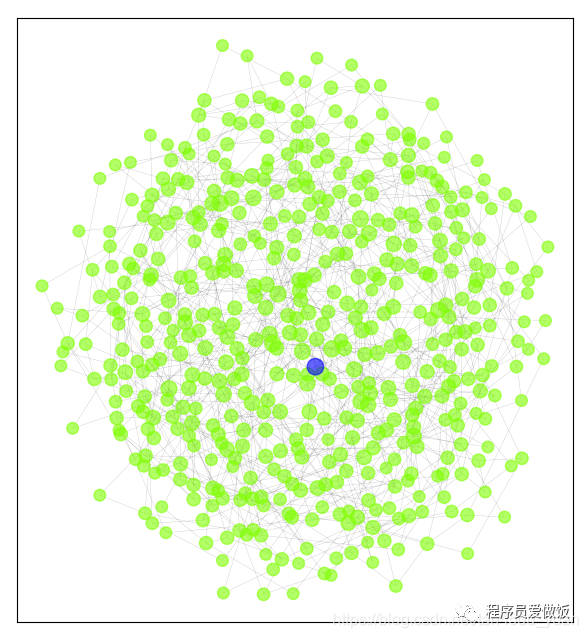小世界网络图

上面这张图片是我绘制的社交关系图，其中蓝色节点代表的是度最高的节点，就是社交关系最复杂的节点。
二、NetWorkx安装
安装方式主要有三种 1.命令行pip 2.pycharm安装 3.官方下载whl文件进行安装
下面我给大家介绍最简单方便的第一种方式吧
1.win+r进入命令行界面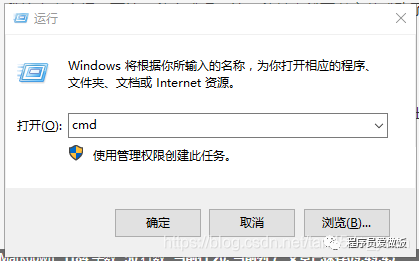在这里插入图片描述

2.输入安装代码
pip install networkx -i http://pypi.douban.com/simple --trusted-host pypi.douban.com

这里更换了豆瓣的镜像源。可以提高下载速度。安装其他包的时候，将networkx改成其他包名即可。
三、NetworkX基础知识
1.创建图
首先我们需要创建一个没有边和节点的图形，说白了就是先拿出一张白纸，我们准备在白纸上作画了。
import networkx as nx
G = nx.Graph()#无多重边无向图
G = nx.DiGraph()#无多重边有向图
G = nx.MultiGraph()#有多重边无向图
G = nx.MultiDiGraph()#有多重边有向图

可以创建四种图形，无多重边无向图、无多重边有向图、有多重边无向图、有多重边有向图。常用的就是第一种图了
2.添加节点
这一步的作用就是在图中添加节点，我们可以一次添加一个节点，也可以添加一个节点列表
G.add_node(1)#添加节点1

两个命令是不一样的需要注意一下哦
3.添加边
当然边也可以单个添加和多个添加
G.add_edge('x', 'y')   # 添加一条边起点为x,终点为y
G.add_edges_from([(1, 2), (1, 3), (2, 3)])   # 添加多条边

下面我们来看一下当前效果
import networkx as nx
import matplotlib.pyplot as plt

G = nx.Graph()  # 无多重边无向图
G.add_nodes_from([2, 3, 4, 5, 6])  # 添加节点2，3
G.add_edges_from([(1, 2), (4, 5), (5, 6), (2, 4)]);
G.add_edges_from([(1, 2), (1, 3), (2, 3)])  # 添加多条边
nx.draw(G, with_labels=True);
plt.show()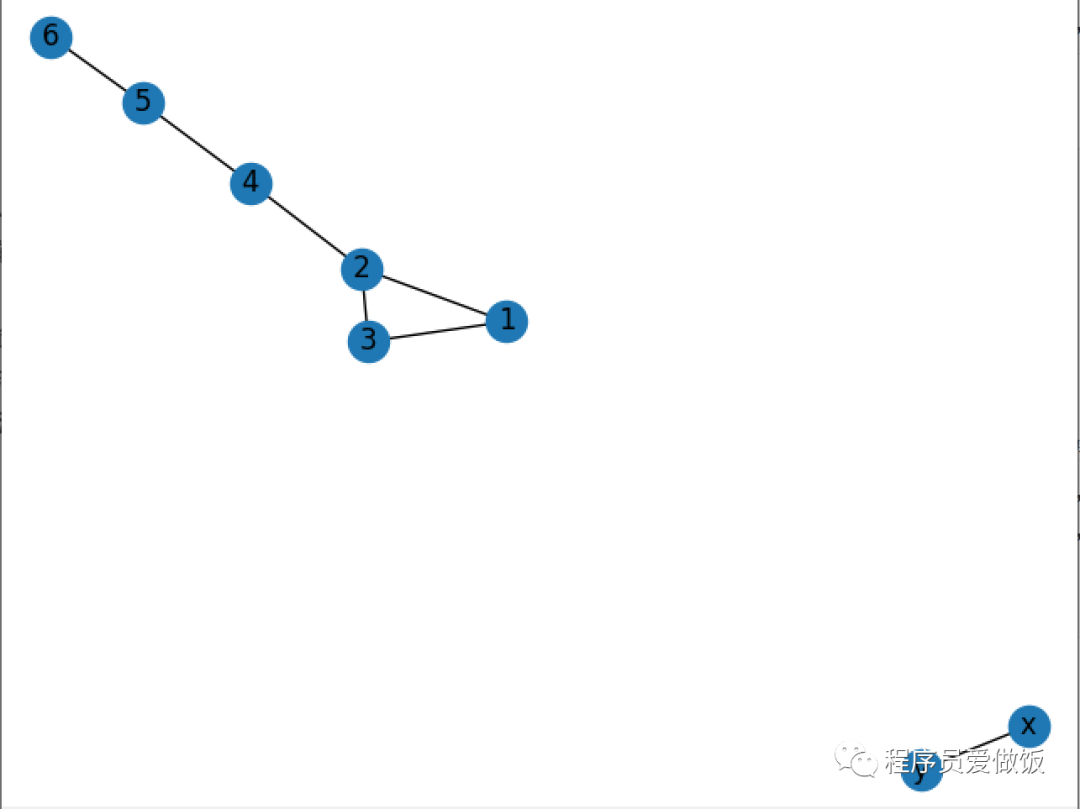4.给图中的节点和边添加属性
运行样式：
- node_size:  指定节点的尺寸大小(默认是300)
- node_color:  指定节点的颜色 (默认是红色，可以用字符串简单标识颜     色，例如'r'为红色，'b'为绿色等)
- node_shape:  节点的形状（默认是圆形，用字符串'o'标识）
- alpha: 透明度 (默认是1.0，不透明，0为完全透明)
- width: 边的宽度 (默认为1.0)
- edge_color: 边的颜色(默认为黑色)
- style: 边的样式(默认为实现，可选：solid|dashed|dotted,dashdot)
- with_labels: 节点是否带标签（默认为True）
- font_size: 节点标签字体大小 (默认为12)
- font_color: 节点标签字体颜色（默认为黑色）
- pos: 布局

运用布局：
circular_layout：节点在一个圆环上均匀分布
random_layout：节点随机分布
shell_layout：节点在同心圆上分布
spring_layout： 用Fruchterman-Reingold算法排列节点（样子类似多中心放射状）
spectral_layout：根据图的拉普拉斯特征向量排列节点
我们需要在nx.draw这行代码里面添加属性。

下面我们来使用一下这些属性，看看会有什么效果。
添加节点属性
import networkx as nx
import matplotlib.pyplot as plt

G = nx.Graph()  # 无多重边无向图
G.add_edges_from([(1, 2), (4, 5), (5, 6), (2, 4),(1,3),(2,4)]);
nx.draw(G, with_labels=True,node_size=200,node_color='#7FFF00')#在这里添加属性，添加颜色和大小
plt.show()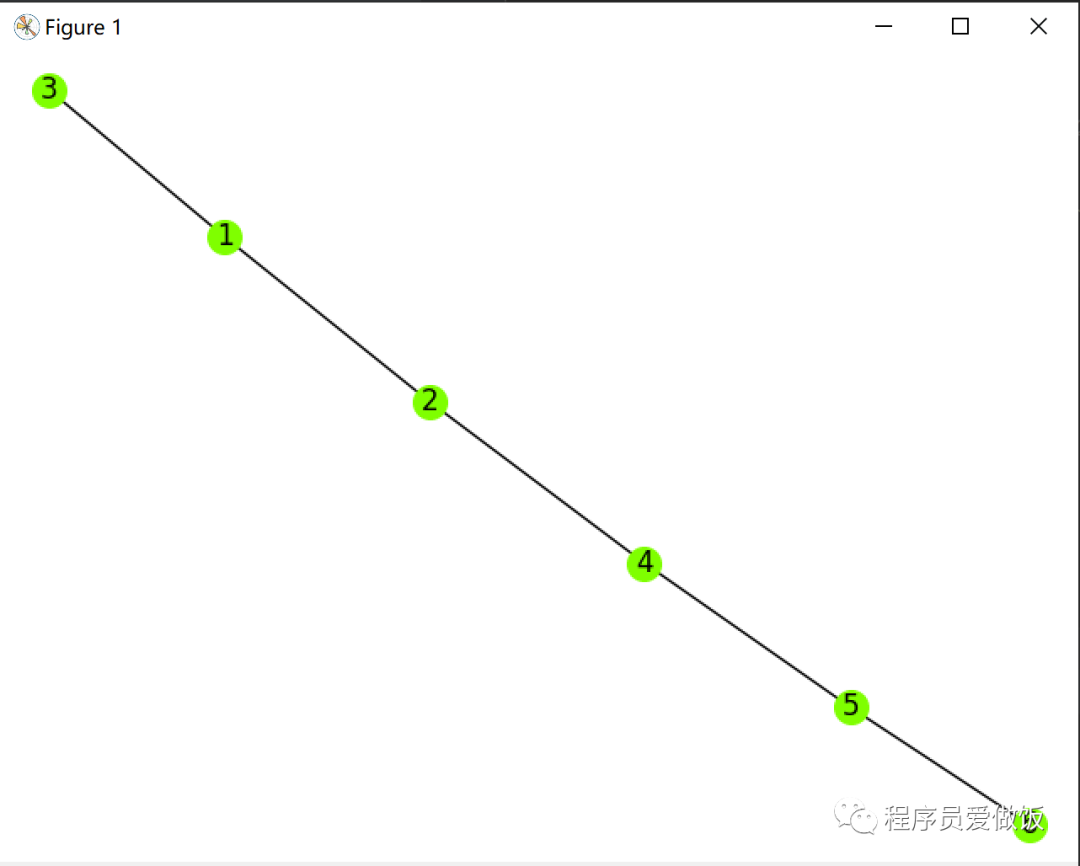添加布局属性
pos =nx.shell_layout(G)
nx.draw(G,with_labels=True,node_size=200,node_color='#7FFF00',pos=pos)

import networkx as nx
import matplotlib.pyplot as plt

G = nx.Graph()  # 无多重边无向图
G.add_edges_from([(1, 2), (4, 5), (5, 6), (2, 4),(1,3),(2,4),(3,6),(3,7),(4,8),(5,9),(7,10),(1,10)]);
pos =nx.shell_layout(G)
nx.draw(G,with_labels=True,node_size=200,node_color='#7FFF00',pos=pos)
plt.show()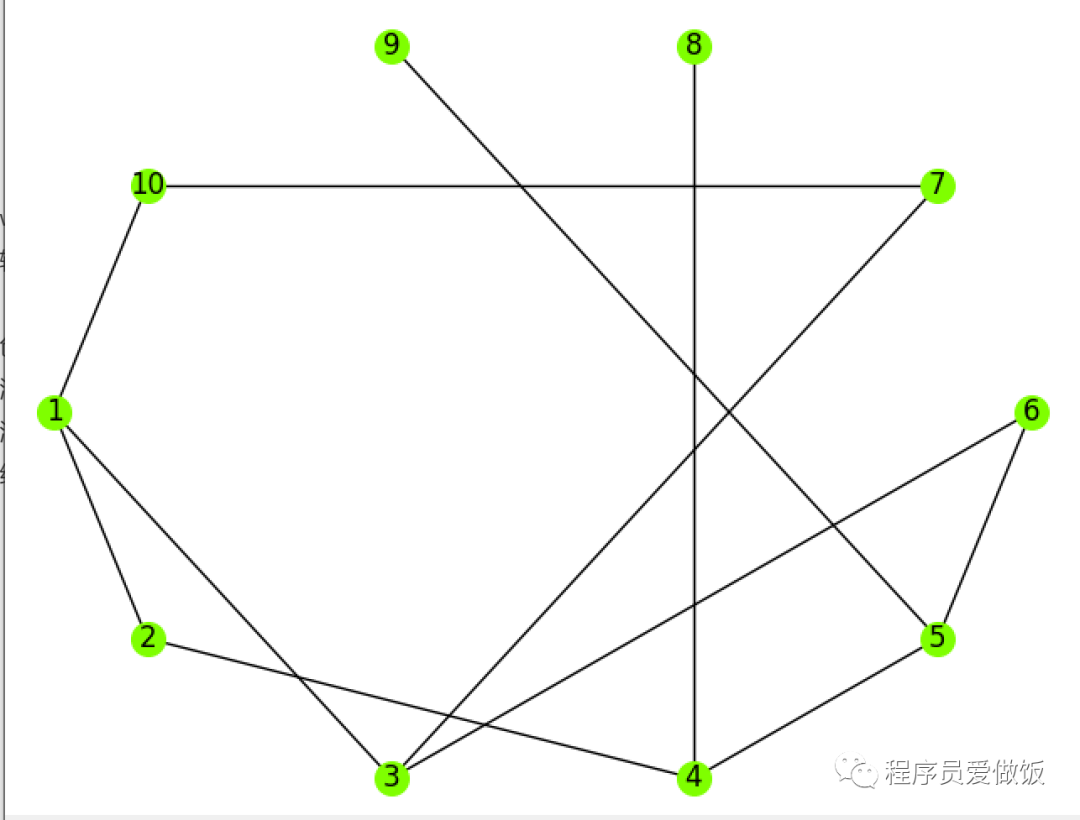我们刚才用的属性是节点在同心圆上分布，效果如上图。还有其他分布方式大家可以试一下。
给节点添加不同的颜色
import networkx as nx
import matplotlib.pyplot as plt

G = nx.Graph()  # 无多重边无向图
color=['#7FFF00','#0000ff','#9999ff','#ff00ff']
pos =nx.shell_layout(G)
nx.draw(G, with_labels=True,node_size=200,node_color=color,pos=pos)
plt.show()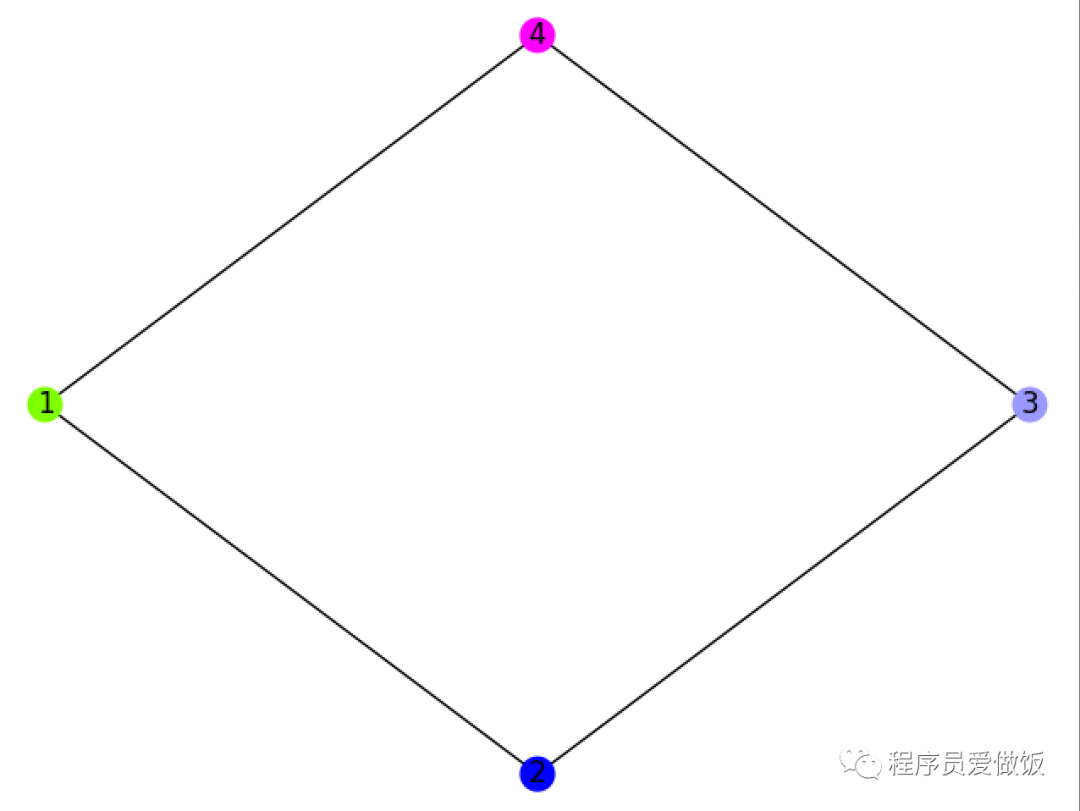不同节点不同颜色

我们还可以给每个节点设置不同的颜色。当然大小也可以，这里自由发挥就好了。
5.样例实现
我们用了两种不同的节点分布方式，效果如下。
import networkx as nx
import matplotlib.pyplot as plt
net_grid = nx.Graph()
# nodes
list_net_nodes = [1, 2, 3, 4, 5, 6, 7, 8, 9, 10, 11, 12, 13, 14, 15, 16, 17, 18, 19, 20]
# edges
list_net_edges = [(1, 3), (3, 5), (5, 4), (4, 2), (2, 6),
(5, 7), (5, 8), (8, 6),
(7, 9), (8, 9), (6, 10),
(9, 11), (10, 12), (10, 13),
(11, 14), (12, 14), (12, 15),
(14, 16), (15, 16), (15, 17),
(16, 18), (17, 19),(12, 2),(12, 1),
(18, 20), (19, 7),(19, 2),(19, 1),(19, 5)]
pos = nx.random_layout(net_grid)#随机分布
nx.draw_networkx_nodes(net_grid, pos=pos, node_color='#ff0000', node_size=200, alpha=0.6)#点的样式
nx.draw_networkx_edges(net_grid, pos=pos, width=0.5, alpha=0.4)#边的样式
plt.show()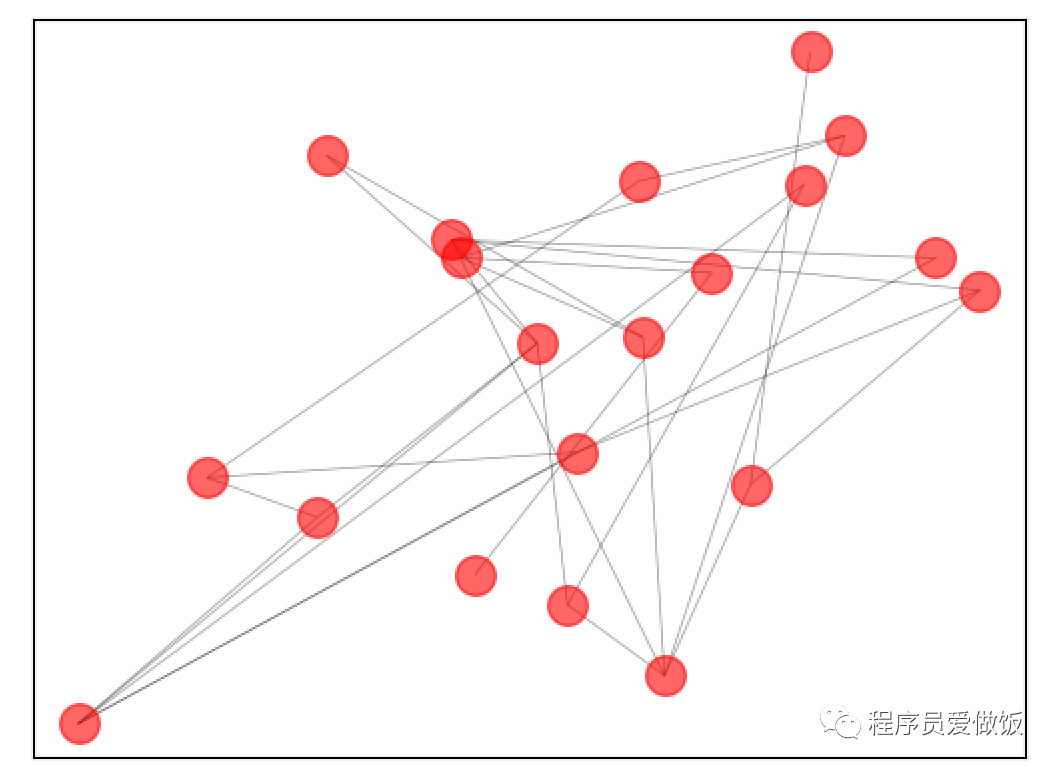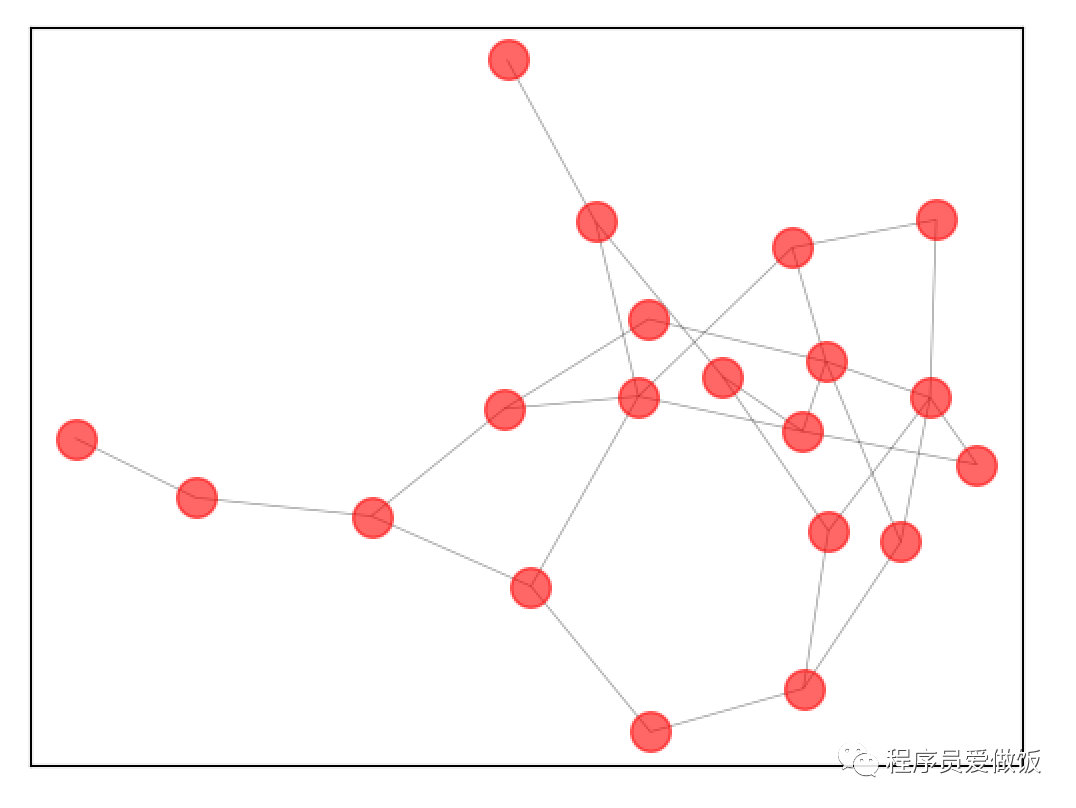学到这里基本的图就会画了，下面学习一下通过数据集进行绘制绘制网络图
6.导入数据进行绘图
利用football数据集绘制社交关系图，
import networkx as nx
import matplotlib.pyplot as plt
net_grid = nx.Graph()
#构建函数，提取出数据集里的边和点
nodedata= H.nodes;
eagedata=H.edges;
return nodedata,eagedata
#引用函数得到边和点
pos = nx.random_layout(net_grid)#随机分布图
nx.draw_networkx_nodes(net_grid, pos=pos, node_color='#7FFF00', node_size=150, alpha=0.7)#点的样式
nx.draw_networkx_edges(net_grid, pos=pos, width=0.3, alpha=0.2)#边的样式
plt.show()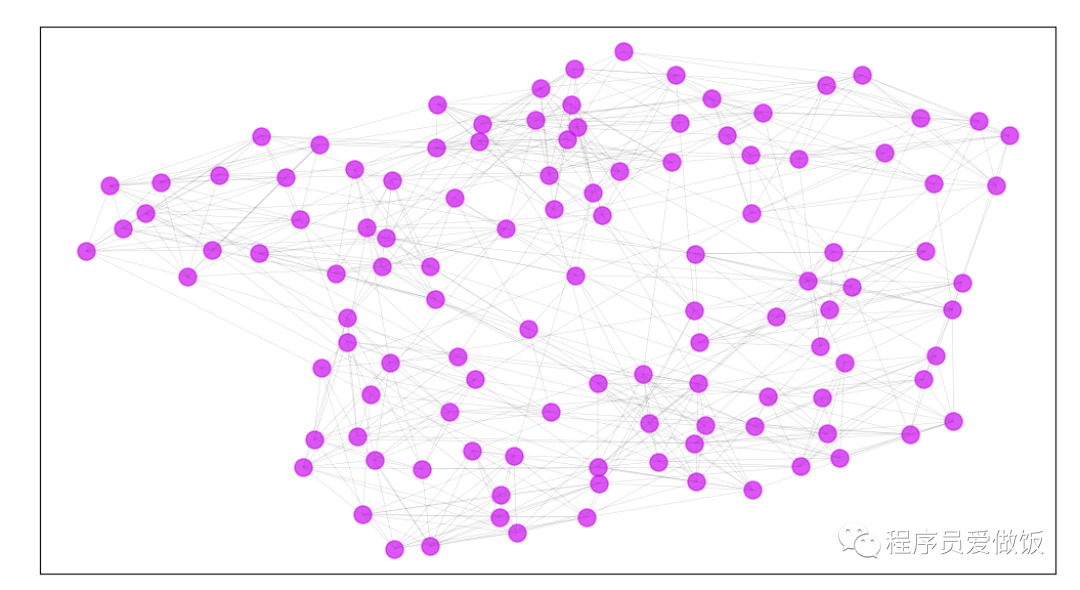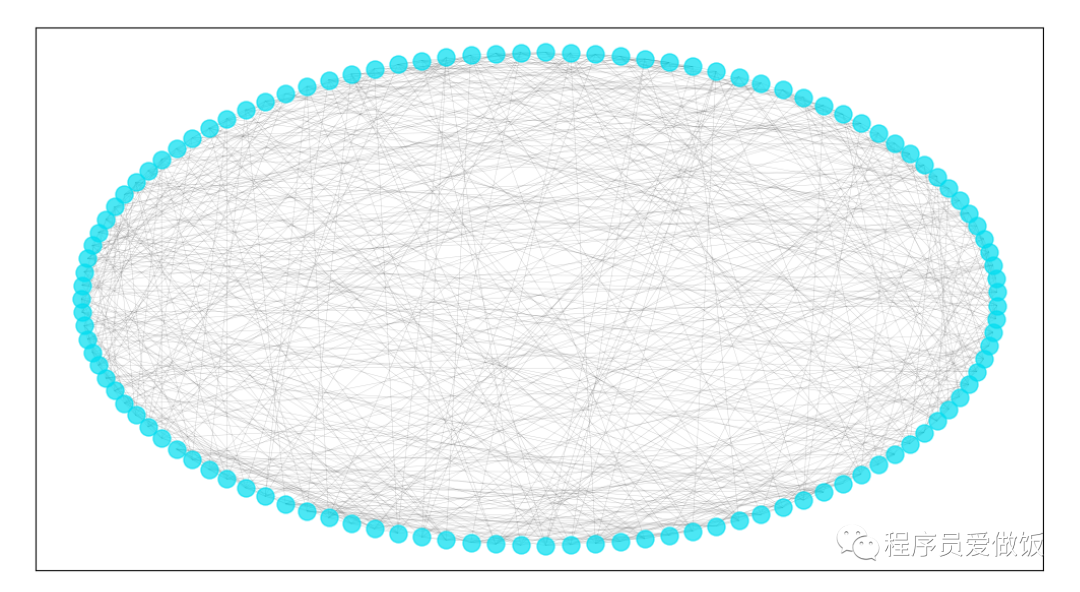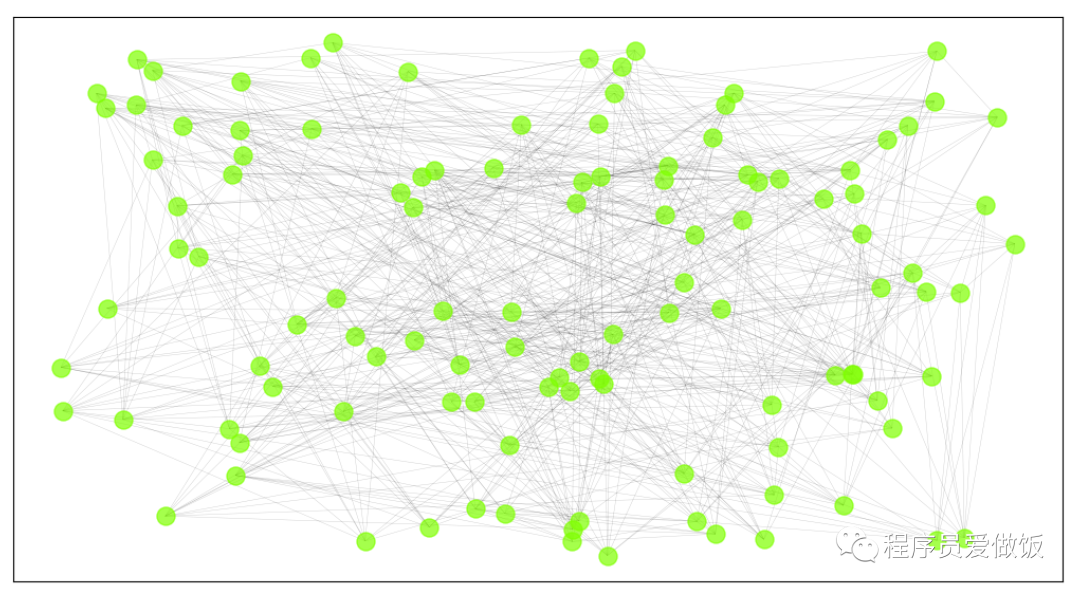上面三张图片是利用不同的排列方式进行排列的。Networkx还有很多强大的功能，大家可以继续深挖，这里为大家提供一个入门参考，感谢大家的支持。如果大家感觉Networkx不能满足大家的需求，绘制网络图的python库还有DGL，PyG。
如果大家需要可以私信我

展开全文css 数据可视化 编程语言 人工智能
• ## python生成语谱图

千次阅读 2019-05-07 15:43:48
时域分析对语音信号的频率特性没有直观的了解，频域特性中又没有语音信号随时间的变化关系。而语谱综合了时域和频域的优点，明显的显示出了语音频谱随时间的变化情况、语谱的横轴为时间，纵轴为频率，任意给定...
前言
本文的文字及图片来源于网络,仅供学习、交流使用,不具有任何商业用途,如有问题请及时联系我们以作处理。
PS：如有需要Python学习资料的小伙伴可以加点击下方链接自行获取
python免费学习资料以及群交流解答点击即可加入
语音的时域分析和频域分析是语音分析的两种重要方法，但是都存在着局限性。时域分析对语音信号的频率特性没有直观的了解，频域特性中又没有语音信号随时间的变化关系。而语谱图综合了时域和频域的优点，明显的显示出了语音频谱随时间的变化情况、语谱图的横轴为时间，纵轴为频率，任意给定频率成分在给定时刻的强弱用颜色深浅来表示。颜色深的，频谱值大，颜色浅的，频谱值小。语谱图上不同的黑白程度形成不同的纹路，称之为声纹，不同讲话者的声纹是不一样的，可用作声纹识别。
下面是在python中绘制语谱图：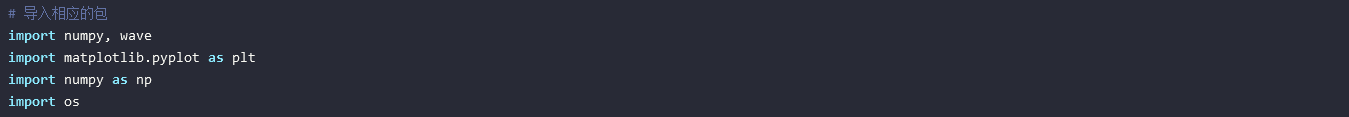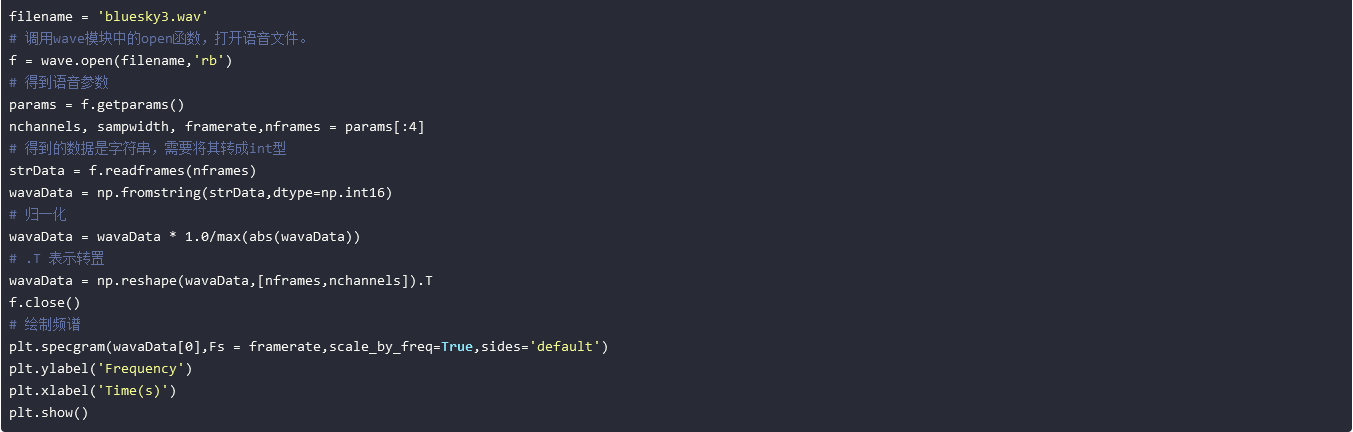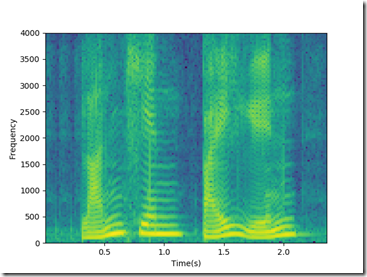展开全文• 求大佬指点 做公司的连锁董事网络，一个公司对应多个董事，如果两家公司有相同的董事则设两家公司的关系为1，否则为0。需要生成公司与公司之间的关系矩阵。这个要怎么弄？ </p>有问必答
1. 创建一个图
import networkx as nx
g = nx.Graph()
g.clear() #将图上元素清空
所有的构建复杂网络图的操作基本都围绕这个g来执行。
2. 节点
节点的名字可以是任意数据类型的，添加一个节点是
添加一组节点，就是提前构建好了一个节点列表，将其一次性加进来，这跟后边加边的操作是具有一致性的。
or
a = [2,3]
g.nodes() #可以将以上5个节点打印出来看看
加一组从0开始的连续数字的节点
H = nx.path_graph(10)
删除节点
与添加节点同理
g.remove_node(node_name)
g.remove_nodes_from(nodes_list)
3. 边
边是由对应节点的名字的元组组成，加一条边
e = (2,3)
加一组边
通过nx.path_graph(n)加一系列连续的边
n = 10
H = nx.path_graph(n)
删除边
同理添加边的操作
g.remove_edge(edge)
g.remove_edges_from(edges_list)
4. 查看图上点和边的信息
g.number_of_nodes() #查看点的数量
g.number_of_edges() #查看边的数量
g.nodes() #返回所有点的信息(list)
g.edges() #返回所有边的信息(list中每个元素是一个tuple)
g.neighbors(1) #所有与1这个点相连的点的信息以列表的形式返回
g #查看所有与1相连的边的属性，格式输出：{0: {}, 2: {}} 表示1和0相连的边没有设置任何属性（也就是{}没有信息），同理1和2相连的边也没有任何属性
method
explanation
Return True if the graph contains the node n.
Return True if n is a node, False otherwise.
Return True if the edge (u,v) is in the graph.
Return the number of nodes in the graph.
Return the number of nodes in the graph.
Return the number of nodes.
Graph.degree([nbunch, weight])
Return the degree of a node or nodes.
Graph.degree_iter([nbunch, weight])
Return an iterator for (node, degree).
Graph.size([weight])
Return the number of edges.
Return the number of edges between two nodes.
Return a list of nodes with self loops.
Return a list of selfloop edges.
Return the number of selfloop edges.
5. 图的属性设置
为图赋予初始属性
g = nx.Graph(day="Monday")
g.graph # {'day': 'Monday'}
修改图的属性
g.graph['day'] = 'Tuesday'
g.graph # {'day': 'Tuesday'}
6. 点的属性设置
print g.node['benz'] # {'fuel': '1.5L', 'money': 10000}
print g.node['benz']['money'] # 10000
print g.nodes(data=True) # data默认false就是不输出属性信息，修改为true，会将节点名字和属性信息一起输出
7. 边的属性设置
通过上文中对g的介绍可知边的属性在{}中显示出来，我们可以根据这个秀改变的属性
g.clear()
n = 10
H = nx.path_graph(n)
g['color'] = 'blue'
g['weight'] = 4.7
g.edge['weight'] = 4
8. 不同类型的图（有向图Directed graphs , 重边图 Multigraphs）
Directed graphs
DG = nx.DiGraph()
print DG.out_degree(1) # 打印结果：2 表示：找到1的出度
print DG.out_degree(1, weight='weight') # 打印结果：0.8 表示：从1出去的边的权值和，这里权值是以weight属性值作为标准，如果你有一个money属性，那么也可以修改为weight='money'，那么结果就是对money求和了
print DG.successors(1) # [2,4] 表示1的后继节点有2和4
print DG.predecessors(1) #  表示只有一个节点3有指向1的连边
Multigraphs
简答从字面上理解就是这种复杂网络图允许你相同节点之间允许出现重边
MG=nx.MultiGraph()
print MG.degree(weight='weight') # {1: 1.25, 2: 1.75, 3: 0.5}
GG=nx.Graph()
for nbr,edict in nbrs.items():
minvalue=min([d['weight'] for d in edict.values()])
print nx.shortest_path(GG,1,3) # [1, 2, 3]
9. 图的遍历
g = nx.Graph()
print n, nbrs
for nbr,eattr in nbrs.items():
# nbr表示跟n连接的点，eattr表示这两个点连边的属性集合，这里只设置了weight，如果你还设置了color，那么就可以通过eattr['color']访问到对应的color元素
data=eattr['weight']
if data<0.5: print('(%d, %d, %.3f)' % (n,nbr,data))
10. 图生成和图上的一些操作
下方的这些操作都是在networkx包内的方法
subgraph(G, nbunch) - induce subgraph of G on nodes in nbunch
union(G1,G2) - graph union
disjoint_union(G1,G2) - graph union assuming all nodes are different
cartesian_product(G1,G2) - return Cartesian product graph
compose(G1,G2) - combine graphs identifying nodes common to both
complement(G) - graph complement
create_empty_copy(G) - return an empty copy of the same graph class
convert_to_undirected(G) - return an undirected representation of G
convert_to_directed(G) - return a directed representation of G
11. 图上分析
g = nx.Graph()
nx.connected_components(g) # [[1, 2, 3], ['spam']] 表示返回g上的不同连通块
sorted(nx.degree(g).values())
通过构建权值图，可以直接快速利用dijkstra_path()接口计算最短路程
>>> G=nx.Graph()
>>> e=[('a','b',0.3),('b','c',0.9),('a','c',0.5),('c','d',1.2)]
>>> print(nx.dijkstra_path(G,'a','d'))
['a', 'c', 'd']
12. 图的绘制
下面是4种图的构造方法，选择其中一个
nx.draw(g)
nx.draw_random(g) #点随机分布
nx.draw_circular(g) #点的分布形成一个环
nx.draw_spectral(g)
最后将图形表现出来
import matplotlib.pyplot as plt
plt.show()
将图片保存到下来
nx.draw(g)
plt.savefig("path.png")
修改节点颜色，边的颜色
g = nx.cubical_graph()
nx.draw(g, pos=nx.spectral_layout(g), nodecolor='r', edge_color='b')
plt.show()
13. 图形种类的选择
Graph Type
NetworkX Class
简单无向图
Graph()
简单有向图
DiGraph()
有自环
Grap(),DiGraph()
有重边
MultiGraph(), MultiDiGraph()
以上就是本文的全部内容，希望对大家的学习有所帮助，也希望大家多多支持脚本之家。
展开全文• Python将Excel网络关系（两列，id1,id2）转换为邻接矩阵（有向网络和无向网络均可），并画出网络
• 原标题：文本分析之制作网络关系图 Pythonimport networkx as nx import matplotlib.pyplot as plt colors = ['red', 'green', 'blue', 'yellow'] #有向图 DG = nx.DiGraph() #一次性添加多节点，输入的格式为列表 ...
• PyTorch实现“学习使用生成式对抗网络发现跨域关系
• 利用Pajek软件批量处理excel数据，批量生成网络关系图    Pajek软件大家想必都不陌生，但是我很陌生，因为我用它不是进行网络分析，而是用它来画关系图（类似网络图）。但是Pajek软件手工操作时一次只能导入一个...Pajek Excel
• 最近因为数学建模3天速成Python,然后做了一道网络的题，要画网络图。在网上找了一些，发现都是一些很基础的丑陋红点，并且关于网络的一些算法也没有讲，于是自己进http://networkx.github.io/学习了一下。以下仅博...
• 没有一个循环一个function就能直接出的，不信你看—— {r} setwd('d:/Rproject') set.seed(200) App.use("app_data.txt",header = FALSE,sep= ",", colClasses =c("character","character")) g1(App.use)  #...
• 最近忙于学术，公众号更新的有点慢了，在这里给大家个歉，希望大家能继续支持我。...今天给大家带来我写好的脚本，用来分析社会网络关系。这个我没有用到gephi或者其他的工具，是我用python纯脚...
• 说到Python 协程就会想到，进程和线程，当然更离不开生成器。 一 进程简介 一个程序的执行实例就是一个进程。每一个进程提供执行程序所需的所有资源。（进程本质上是资源的集合） 一个进程有一个虚拟的地址空间、...线程 协程 进程
• Python当前人气暴涨。它在DevOps，数据科学，Web开发和安全领域均有使用。但是在速度方面没有赢得美誉。这里有关于Python比较其他语言如，Java, C#, Go, JavaScript, C++进行性能对比，其中Python是最慢的。包含了...
• 点越大表示出现的次数越多）'%fn[:-4],fontsize=12)#-1，1字体位置 plt.savefig('%s-=人物关系图.png'%fn[:-4],dpi=120)#单个像素的DPI 2020年6月16日更新： 因为源代码不在这台电脑上，只针对其中不明确的地方做个...
• 前言本文的文字及图片来源于网络,仅供学习、交流使用,不具有任何商业用途,版权归原作者所有,如有问题请及时联系我们以作处理。作者： 想读博的针针PS：如有需要Python学习资料的小伙伴可以加点击下方链接自行获取...
• igraph提供了R和C语言程序包，以及Python和Ruby语言扩展，它包括的功能包括：网络可视化传统图论算法：最小生成树，网络流等复杂网络处理算法：随机网络模型，网络处理（k-cores, PageRank, betweenness, ...
• ## python社交网络图

千次阅读 2020-04-02 09:36:09
社交网络图 nx.circular_layout()指定网络图节点的布局方式 nx.draw_networkx_nodes()绘制网络图的节点 nx.draw_networkx_edges()绘制网络的边 nx.draw_networkx_labels()为节点添加标签 import networkx as nx ...可视化
• Networkx2Turi()就是这么生成的。 pos代表每个点的位置，一般来说很难定义这个坐标。 node_color每个点的颜色，此时的y代表黄色； with_labels每个顶点是否带文字内容； node_size每个点的大小。 . ...可视化 network networkx turicreate
• 左：两方社区关系图（圆形节点表示三个社区，正方形节点代表网络的节点），右：AGM生成网络，社区关系图在左侧 当我们使用适合于实际网络的 合成网络时，合成网络具有与真实网络非常相似的特征...模型
• 今天给大家带来我一个脚本，用来分析社会网络关系。 这个我没有用到gephi或者其他的工具，是我用python纯脚本运行出来的。简单的实现了封装，大家有兴趣可以下载下脚本，运行下。 原理知识 我就简单说下原理吧，...
• 在本章中，我们将学习如何在Python生成图形和图表，同时将使用函数和面向对象的方法来可视化数据。 Python中常用的一些可视化数据包括以下几种。 Matplotlib。 Seaborn。 ggplot。 Geoplotlib。 Bokeh。 Plotly。...
• 本文改编自CSDN，作者ZhouMu，原文链接如下：https://blog.csdn.net/zhoujinyu0713/article/details/10163037?utm_source=tuicool本文的主要工作是，说明拓扑序的含义及其在生成DAG时的妙用，并给原文代码标注释。...
• 生成对抗网络 图像生成Machines are generating perfect images these days and it’s becoming more and more difficult to distinguish the machine-generated images from the originals.如今，机器正在生成完美...java 机器学习 计算机视觉
• Python神经网络编程 之前说过要转深度学习这块的，导师push的紧，只能摸石头过河，一方面阅读一些相关书籍，一方面跑一些模型修改源码去解决实际的问题。今天给大家介绍一本Python神经网络编程，今年4月份出版的：...深度学习
• Python】Matplotlib画图（十）——基于networkx画关系网络图
• 使用从数据库中导出的论文、专利数据，做作者/专利...生成合作网络代码主函数： if __name__ =='__main__' : co_list = [ ["AA | BB | CC | DD",2019], ["EE | BB | FF ",2018], ["AA | GG | FF | HH | KK",2019],算法 列表...

# python生成网络关系图python 订阅